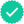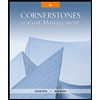Question

Choose the best answer for each of the following multiple-choice questions.
1. Cost-volume-profit analysis includes some simplifying assumptions. Which of the
following is not one of these assumptions?
a. Cost and revenues are predictable.
b. Cost and revenues are linear over the relevant range.
c. Changes in beginning and ending inventory levels are insignificant in amount.
d. Sales mix changes are irrelevant.

2. The term relevant range, as used in cost accounting, means the range
a. over which costs may fluctuate
b. over which cost relationships are valid
c. of probable production
d. over which production has occurred in the past 10 years
3. How would the following be used in calculating the number of units that must be
sold to earn a targeted operating income?

 Price per unit Targeted operating income Denominator Numerator Numerator Numerator Not used Denominator Numerator Denominator

4. Information concerning Korian Corporation’s product is as follows:

 Sales \$300,000 Variable costs 240,000 Fixed costs 40,000

Assuming that Korian increased sales of the product by 20 percent, what should the
operating income be?
a. \$20,000
b. \$24,000
c. \$32,000
d. \$80,000
5. The following data apply to McNally Company for last year:

 Total variable costs per unit \$3.50 Contribution margin/sales 30% Break-even sales (present volume) \$1,000,000

McNally wants to sell an additional 50,000 units at the same selling price and contribution
margin. By how much can fixed costs increase to generate additional profit equal to 10
percent of the sales value of the additional 50,000 units to be sold?
a. \$50,000
b. \$57,500
c. \$67,500
d. \$125,000
6. Bryan Company’s break-even point is 8,500 units. Variable cost per unit is \$140,
and total fixed costs are \$297,500 per year. What price does Bryan charge?
a. \$140
b. \$35
c. \$175
d. Cannot be determined from the above data

Expert SolutionStudents who’ve seen this question also like:Your question is solved by a Subject Matter ExpertWant to see this answer and more?
Experts are waiting 24/7 to provide step-by-step solutions in as fast as 30 minutes!*
*Response times may vary by subject and question complexity. Median response time is 34 minutes for paid subscribers and may be longer for promotional offers.8+ million solutions